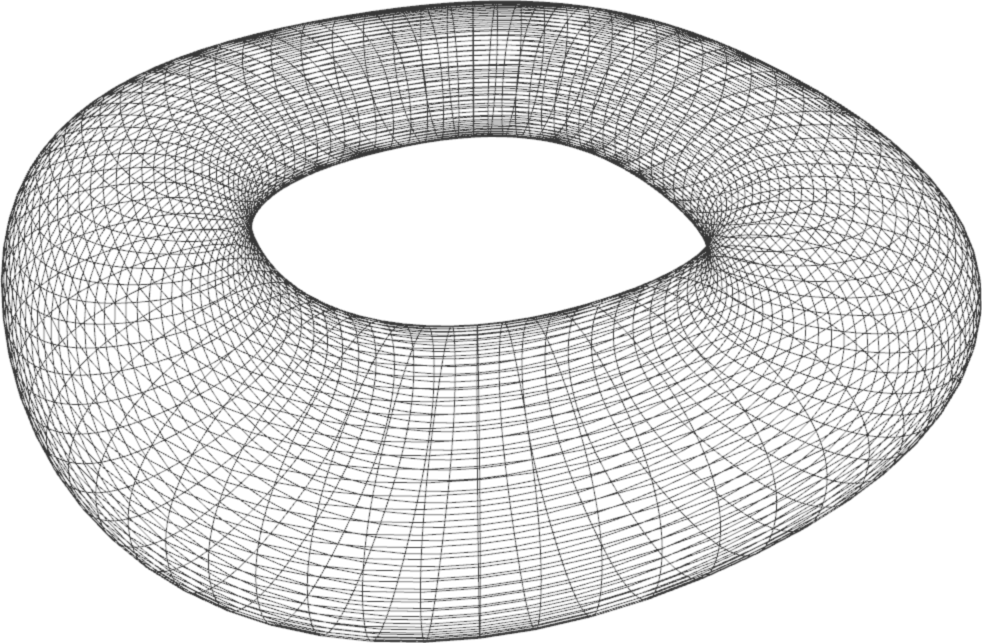# TP7 : B-spline Surfaces

25 March 2016 in

## Code

Do

git pull


or, if you don’t have the local repo,

git clone https://github.com/bbrrck/geo-num-2016.git


As usual, test by

cd TP7/
mkdir build
cd build
cmake ..
make


To test the viewer, try running

./geonum_TP7 simple


If everything goes well, you should see a cube. The viewer can be controlled with the mouse: click and drag to rotate, scroll to zoom (also works with pageup/pagedown keys).

In case the SimpleViewer does not work, you can still export and visualise the surfaces via the python script, included in plots/.

## B-spline curves revisited

Back in TP3, we were working with B-spline curves. A B-spline curved is defined by the following:

## Tensor product B-spline surfaces

A B-spline surface is defined via

The B-spline surface is defined for $(u,v) \in [u_k,u_{m+1}] \times [v_l,v_{n+1}]$ as

## Surface Patches

Recall that a B-spline curve is made from many smaller pieces, defined over parameter intervals $[t_i,t_{i+1}]$. Analogically, a B-spline surface consists of $(m-k+1) \times (n-l+1)$ patches, each defined over a parameter rectangle $[u_i,u_{i+1}] \times [v_j,v_j+1]$ :

Each patch needs to be evaluated individually, then stored in one big matrix (per coordinate).

## Evaluation

To evaluate a point on a B-spline surface, use the same principle as in the last TP: first, evaluate in the $u$ direction $n+1$ times; then use the computed points as the control polygon for a curve in the $v$ direction. As always, this can be done iteratively or recursively. A recursive implementation of the DeBoor’s algorithm is included in todo.h; however, it is strongly advised to use you own (from TP3).

We will use the same paradigm as in the previous TP, i.e. the points will be stored coordinate-wise; in matrices X, Y, Z (or netX, netY, netZ for the control net).

## Algorithm

The implementation of a B-spline surface can be summarized in the following pseudocode.

# loop over all patches
for i in (k,k+1,...,m) :
for j in (l,l+1,...,n) :
# loop over the samples of the current patch
# defined over [u_i,u_{i+1}] x [v_j,v_{j+1}]
for u in uniform_sampling( u_{i}, u_{i+1}, number_of_samples ) :
for v in uniform_sampling( v_{j}, v_{j+1}, number_of_samples ) :
compute_S(u,v) # a surface point on the current patch


## ToDo

1. Implement the evaluation of B-spline surfaces.
2. Modify the knot vectors for the simple dataset. Experiment with various configurations. How does the surface change?
3. [Bonus] A NURBS surface (Non-Uniform Rational B-Spline) can be used to represent a sphere, the same way we used a NURBS curve to represent the unit circle in TP3. Modify your implementation of B-spline surfaces in order to compute a NURBS surface. The control points and weights for representing a sphere can be found in Representing a Circle or a Sphere with NURBS by David Eberly.